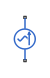# AC Current Source

Ideal sinusoidal current source

## Library

Electrical Sources

•## Description

The AC Current Source block represents an ideal current source that maintains sinusoidal current through it, independent of the voltage across its terminals.

The output current is defined by the following equation:

`$I={I}_{0}\cdot \mathrm{sin}\left(2\pi \cdot f\cdot t+\phi \right)$`

where

 I Current I0 Peak amplitude f Frequency φ Phase shift t Time

The positive direction of the current flow is indicated by the arrow.

Note

For Release 2012b and earlier, the unit definition for Hz was `rev/s`, whereas in R2013a it was changed to be `1/s`, in compliance with the SI unit system. For this block it means that you must specify frequency in units of `Hz` or directly convertible to Hz, such as `1/s`, `kHz`, `MHz` and `GHz`. In 2012b and earlier you could also specify frequency in angular units (such as `rad/s` or `rpm`), but this is no longer possible because the internal equation of the block now uses the 2π conversion factor to account for the `1/s` unit definition. If you use this block in a model created prior to R2013a, update it by using the Upgrade Advisor. For more information, see the R2013a Release Notes.

## Parameters

Peak amplitude

Peak current amplitude. The default value is `1` A.

Phase shift

Phase shift in angular units. The default value is `0`.

Frequency

Current frequency, specified in Hz or units directly convertible to Hz (where Hz is defined as `1/s`). For example, `kHz` and `MHz` are valid units, but `rad/s` is not. The default value is `60` Hz.

## Ports

The block has two electrical conserving ports associated with its terminals.

## Version History

Introduced in R2007a